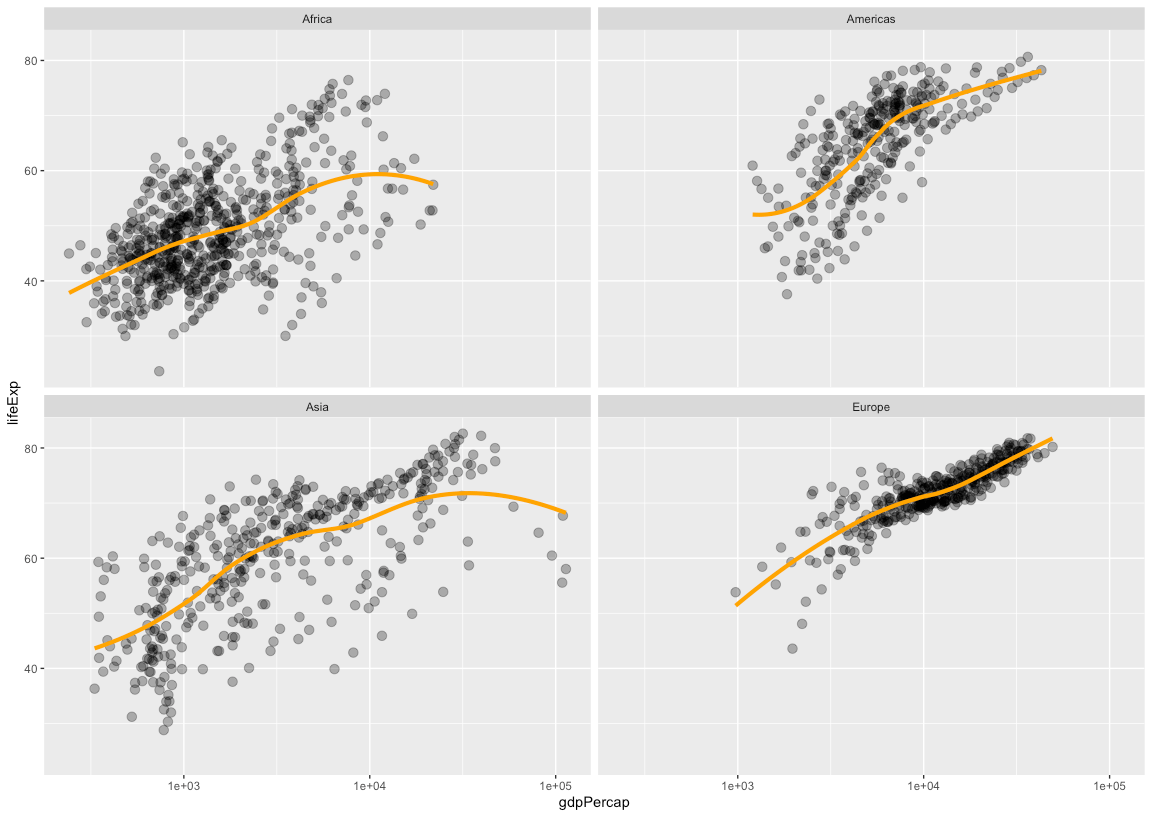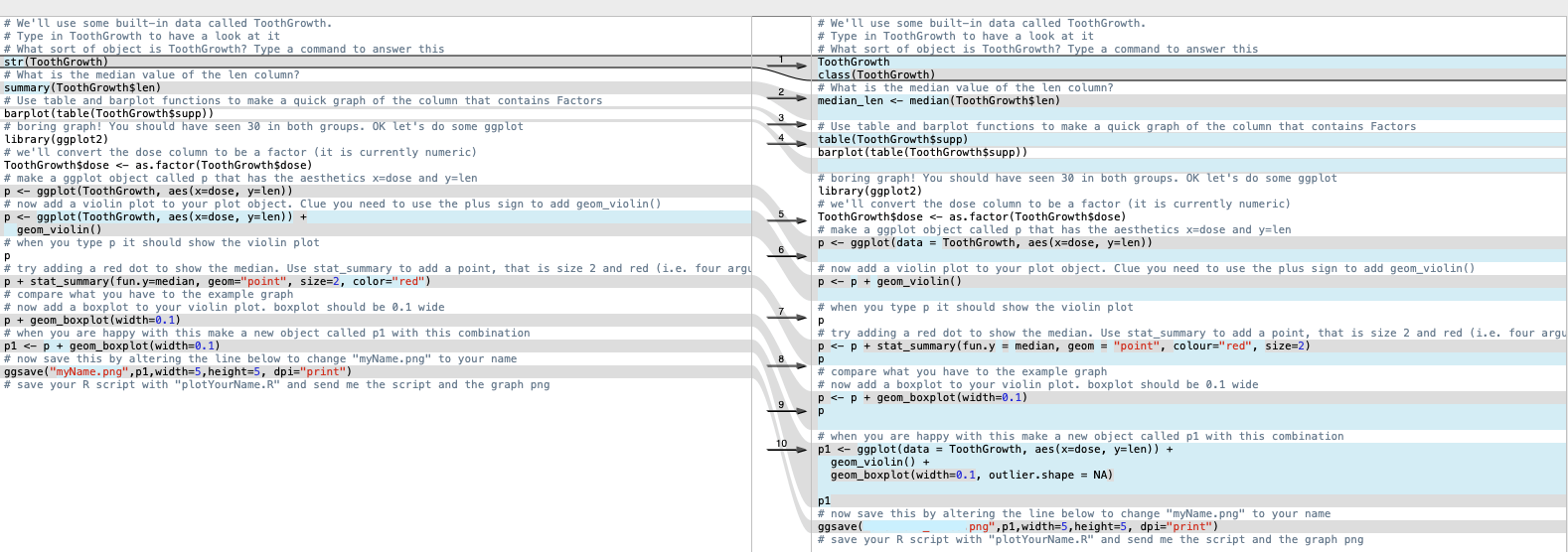# Get Better: R for absolute beginners

Want to share your content on R-bloggers? click here if you have a blog, or here if you don’t.

As part of the series on development of early career researchers in the lab, we spent three sessions over three weeks learning the basics of R.

In my book “The Digital Cell”, I advocate R as the main number-crunching software but the R literacy in my lab is actually quite mixed. In order to know how to pitch the training, I conducted a quick poll in our lab Slack workspace to find out what R skills people in the lab already had. I asked:

My R skills be like…

1. What’s R?
2. I’ve used it but didn’t know what I was doing
3. I’ve taken a class/course
4. I can write an R script
5. I am an R jedi, what can I teach you?

No-one answered 4 or 5, and one person answered 1, so this meant we needed to start from the basics.

I used the excellent STAT545 course materials as a framework and took examples from there. The three sessions were 1) the very basics, 2) dataframes and ggplot, 3) loading in real data, calculations and making a figure. Each session was approx. one hour in a room together with our laptops, followed by a homework exercise that was marked before the next session. Since some people voted 1 or 2 in the poll, session 1 was required. Even the people who voted 3, said that session 1 was a useful reminder, so it might be good to just start here regardless.

Details of the sessions are below and assume you are proficient in R enough to guide newbies through the exercises.

### First session: the very basics

I explained what R and RStudio are. We ironed out people’s installation issues with the software before moving on to packages. As an example, we installed dplyr.

```install.packages("dplyr", dependencies = TRUE)
```

We next covered assignment, objects and functions. Working from the console, we talked about each line:

```x <- seq(1,10)
date() # no arguments
objects()
ls()
rm(list = ls()) # or click broom
```

The discussion about objects and the broom in RStudio brought us onto what the different windows in the IDE are used for. We set up an R project and discussed the working directory.

```getwd()
```

Still working in the console, we went through the following commands to save a simple file and generate a basic plot

```a <- 2
b <- -3
sig_sq <- 0.5
x <- runif(40)
y <- a + b * x + rnorm(40, sd = sqrt(sig_sq))
avg_x <- mean(x)
write(avg_x, "avg_x.txt")
plot(x, y)
abline(a, b, col = "purple")
```

We ran through lines 5, 8 and 9 a few times to understand the rnorm function.

We talked about the History tab and how to rerun commands, either in the console or by moving them to an R script.

We made an R script based on the plot that we’d made and discussed the value of commenting. We discussed reproducibility and what our goal is. To have a dataset and to use a script to reproducibly generate the analysis and output. This was a good place to stop.

#### Homework 1

This was the first homework I set (scroll down the page for the answers).

```#first we will set the same seed, 1234
set.seed(1234)
# make a vector called y of 100 random numbers from a normal distribution # find the 10th value in the vector y (do this using a command) # check the help to see what the mean of y should be (default value of the command you used) # find the actual mean of the vector y # make a vector called x of 100 integers using every other number (odd numbers). Hint: first = 1, last = 199 # make a scatter plot of y versus x # add an orange line at y = 0 # make a histogram of y # This should be line 19. The End
```

To help people check their work, I sent them the plots. Their task was to fill in the blanks in the above R script and submit it back to me to see if I could run it.

### Second session: data frames and ggplot

In this session we concentrated on working with data frames and making some nice looking plots using ggplot. There’s no need to reinvent the wheel here, this session is straight from STAT545 and uses the gapminder library.

By executing one line after another and talking about what was happening, we could work through this exercise together to make some progress and finish with plots, which gave a sense of achievement.

```install.packages("gapminder")
library(gapminder)
str(gapminder)
# we also installed the tidyverse - some people had problems
library(tidyverse) # looking at gapminder
class(gapminder)
gapminder
tail(gapminder)
names(gapminder)
ncol(gapminder)
nrow(gapminder)
length(gapminder)
dim(gapminder)
summary(gapminder) # doing some plots in base R
plot(lifeExp ~ year, gapminder)
plot(lifeExp ~ gdpPercap, gapminder)
plot(lifeExp ~ log(gdpPercap), gapminder) # using data frame terminology - dollar signs for columns
summary(gapminder\$lifeExp)
hist(gapminder\$lifeExp)
class(gapminder\$continent)
summary(gapminder\$continent)
levels(gapminder\$continent)
nlevels(gapminder\$continent)
table(gapminder\$continent)
barplot(table(gapminder\$continent)) # using ggplot
p <-ggplot(filter(gapminder, continent != "Oceania"), aes(x = gdpPercap, y = lifeExp))
p <- p + scale_x_log10()
# our background was set up as an object called p
p + geom_point()
p + geom_point(aes(color = continent))
p + geom_point(alpha = (1/3), size = 3) + geom_smooth(lwd = 3, se = FALSE)
p + geom_point(alpha = (1/3), size = 3) + facet_wrap(~ continent) + geom_smooth(lwd = 1.5, se = FALSE, colour = "orange")
```

In the end we have a nice looking set of plots (below). Earlier in the session, trainees see some base R graphics and then a ggplot version which highlights the aesthetics of ggplot.Session 2 output

#### Homework 2

I supplied my R script (above) from the session, so that people could go over it in their own time. As before, the homework task this week was to fill in the blank lines and submit it back to me together with the plot.

```# We'll use some built-in data called ToothGrowth.
# Type in ToothGrowth to have a look at it
# What sort of object is ToothGrowth? Type a command to answer this # What is the median value of the len column? # Use table and barplot functions to make a quick graph of the column that contains Factors # boring graph! You should have seen 30 in both groups. OK let's do some ggplot
library(ggplot2)
# we'll convert the dose column to be a factor (it is currently numeric)
ToothGrowth\$dose <- as.factor(ToothGrowth\$dose)
# make a ggplot object called p that has the aesthetics x=dose and y=len # now add a violin plot to your plot object. Clue you need to use the plus sign to add geom_violin() # when you type p it should show the violin plot
p
# add a red dot to show the median. Use stat_summary to add a point that is size 2 and red (4 arguments) # compare what you have to the example graph
# now add a boxplot to your violin plot. boxplot should be 0.1 wide # when you are happy with this make a new object called p1 with this combination # now save this by altering the line below to change "myName.png" to your name
ggsave("myName.png",p1,width=5,height=5, dpi="print")
# save your R script with "plotYourName.R" and send me the script and the graph png
```Session 2: this should be the output from line 21

### Third session: real data

The aim of the final session was to give people a taste of generating a real figure, using a script and some real data. Again the emphasis was on reproducibility. I reminded them that the raw data should be untouched and that the outputs are disposable, and can be regenerated at any time, including by other people.

To generate some realistic data we used Fiji to analyse the number of puncta in a microscopy movie. We did this part by sharing the movie in a Dropbox folder and then executing a series of steps (in hindsight, this would’ve been better run as a script in Fiji).

Everybody did this in the session by segmenting the image and then running analyse particles and saving the Results window as a CSV file in the data directory of their R project.

This file was selected after line 2 in the following code which we again went through line-by-line.

```require(ggplot2)
file_name <- file.choose()
summary(df1\$Mean)
# build this up
p1 <- ggplot(df1, aes(Slice,Mean)) + geom_point() + stat_summary(fun.y = mean, geom = "point", size=2, colour="orange") + geom_smooth() + labs(x = "Frame", y = "GFP")
p1
p2 <- ggplot(df1, aes(Slice,Area)) + geom_point() + stat_summary(fun.y = mean, geom = "point", size=2, colour="orange") + geom_smooth() + labs(x = "Frame", y = "Area (pixels)")
p2
p3 <- ggplot(df1, aes(Area,Mean)) + geom_point()
p3
# correct scaling
# 1 pixel is 0.069 * 0.069 um
pxSize <- 0.069 * 0.069
df1\$Area_um2 <- df1\$Area * pxSize
p4 <- ggplot(df1, aes(Area_um2,Mean)) + geom_point()
p4
# 5 sec per frame
df1\$Time <- (df1\$Slice - 1) * 5
p5 <- ggplot(df1, aes(Time,Mean)) + geom_point() + stat_summary(fun.y = mean, geom = "point", size=2, colour="orange") + geom_smooth() + labs(x = "Time (s)", y = "GFP")
p5
# count spots per frame
df2 <- as.data.frame(table(df1\$Slice))
# note the column names
names(df2) <- c("Slice","Count")
df2\$Time <- (df2\$Slice - 1) * 5
# doesn't work - why?
str(df2)
df2\$Slice <- as.integer(df2\$Slice)
str(df2)
df2\$Time <- (df2\$Slice - 1) * 5
p6 <- ggplot(df2, aes(Time,Count)) + geom_point() + scale_y_continuous(limits = c(0,100)) + geom_smooth() + labs(x = "Time (s)", y = "Puncta")
p6
```After this we went through a further example using some data from one person in the lab who needed to plot out their results. We also incorporated a function that I had written for bootstrapping confidence intervals as part of this figure. I have not made this part of the session available in the post.

### Wrap up

The lab members found it useful and didn’t even complain about the homework. All of them could see the value in learning a bit of R, so it was rewarding all round.

The people with no prior experience found the final session pretty tough so I don’t think it would be possible to go through this material in fewer sessions.

Of course, the best way to learn is by doing, so the challenge is to get people to persist with R for their number crunching. A simple way is to keep advocating for analysis to be done in R or more drastically, other software could be outlawed. One idea I had was to have a follow up session to work through a real dataset in a hackathon approach with everyone in the room.

Hopefully this has given you some ideas for R training in your lab.